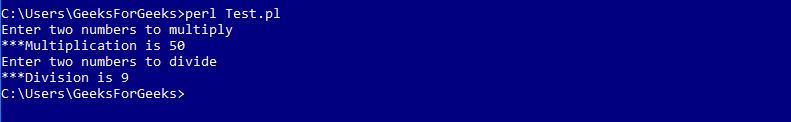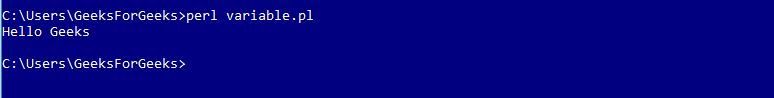# Perl | Modules

A module in Perl is a collection of related subroutines and variables that perform a set of programming tasks. Perl Modules are reusable. Various Perl modules are available on the Comprehensive Perl Archive Network (CPAN). These modules cover a wide range of categories such as network, CGI, XML processing, databases interfacing, etc.

Creating a Perl Module

A modules name must be same as to the name of the Package and should end with .pm extension.

Example : Calculator.pm

 `package` `Calculator; ` ` `  `# Defining sub-routine for Multiplication ` `sub` `multiplication ` `{ ` `    ``# Initializing Variables a & b ` `    ``\$a` `= ``\$_``; ` `    ``\$b` `= ``\$_``; ` `     `  `    ``# Performing the operation ` `    ``\$a` `= ``\$a` `* ``\$b``; ` `     `  `    ``# Function to print the Sum ` `    ``print` `"\n***Multiplication is \$a"``; ` `} ` ` `  `# Defining sub-routine for Division ` `sub` `division ` `{ ` `    ``# Initializing Variables a & b ` `    ``\$a` `= ``\$_``; ` `    ``\$b` `= ``\$_``; ` `     `  `    ``# Performing the operation ` `    ``\$a` `= ``\$a` `/ ``\$b``; ` `     `  `    ``# Function to print the answer ` `    ``print` `"\n***Division is \$a"``; ` `} ` `1; `

Here, the name of the file is “Calculator.pm” stored in the directory Calculator. Notice that 1; is written at the end of the code to return a true value to the interpreter. Perl accepts anything which is true instead of 1

Importing and using a Perl Module

To import this calculator module, we use require or use functions. To access a function or a variable from a module, :: is used. Here is an example demonstrating the same:

Examples: Test.pl

 `#!/usr/bin/perl ` ` `  `# Using the Package 'Calculator' ` `use` `Calculator; ` ` `  `print` `"Enter two numbers to multiply"``; ` ` `  `# Defining values to the variables ` `\$a` `= 5; ` `\$b` `= 10; ` ` `  `# Subroutine call ` `Calculator::multiplication(``\$a``, ``\$b``); ` ` `  `print` `"\nEnter two numbers to divide"``; ` ` `  `# Defining values to the variables ` `\$a` `= 45; ` `\$b` `= 5; ` ` `  `# Subroutine call ` `Calculator::division(``\$a``, ``\$b``); `

Output:Using Variables from modules

Variables from different packages can be used by declaring them before using. Following example demonstrates this
Examples: Message.pm

 `#!/usr/bin/perl ` ` `  `package` `Message; ` ` `  `# Variable Creation ` `\$username``; ` ` `  `# Defining subroutine ` `sub` `Hello ` `{ ` `  ``print` `"Hello \$username\n"``; ` `} ` `1; `

Perl file to access the module is as below
Examples: variable.pl

 `#!/usr/bin/perl ` ` `  `# Using Message.pm package ` `use` `Message; ` ` `  `# Defining value to variable ` `\$Message::username` `= ``"Geeks"``; ` ` `  `# Subroutine call ` `Message::Hello(); `

Output:Using Pre-defined Modules

Perl provides various pre-defined modules which can be used in the Perl programs anytime.
Such as: ‘strict’, ‘warnings’, etc.

Example:

 `#!/usr/bin/perl ` ` `  `use` `strict; ` `use` `warnings; ` ` `  `print``" Hello This program uses Pre-defined Modules"``; `

Output:

`Hello This program uses Pre-defined Modules`

My Personal Notes arrow_drop_upCheck out this Author's contributed articles.

If you like GeeksforGeeks and would like to contribute, you can also write an article using contribute.geeksforgeeks.org or mail your article to contribute@geeksforgeeks.org. See your article appearing on the GeeksforGeeks main page and help other Geeks.

Please Improve this article if you find anything incorrect by clicking on the "Improve Article" button below.

Article Tags :
Practice Tags :

Be the First to upvote.

Please write to us at contribute@geeksforgeeks.org to report any issue with the above content.